Next: Maximum Likelihood Estimate Up: Parameter Estimation for Previous: Parameter Estimation for

## Least Squares Estimate of Parameters

The least squares estimate detailed in  assumes that the observations of the GMRF image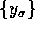obey the modelwhere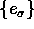is a zero mean correlated noise sequence with variance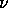and correlation with the following structure: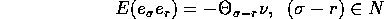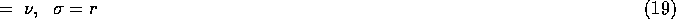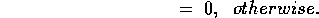The conditional distribution is given in (11). Then, for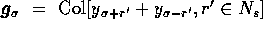, the LSE are: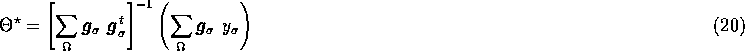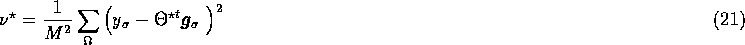where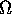is the complete set of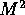pixels, and toroidal wrap-around is assumed.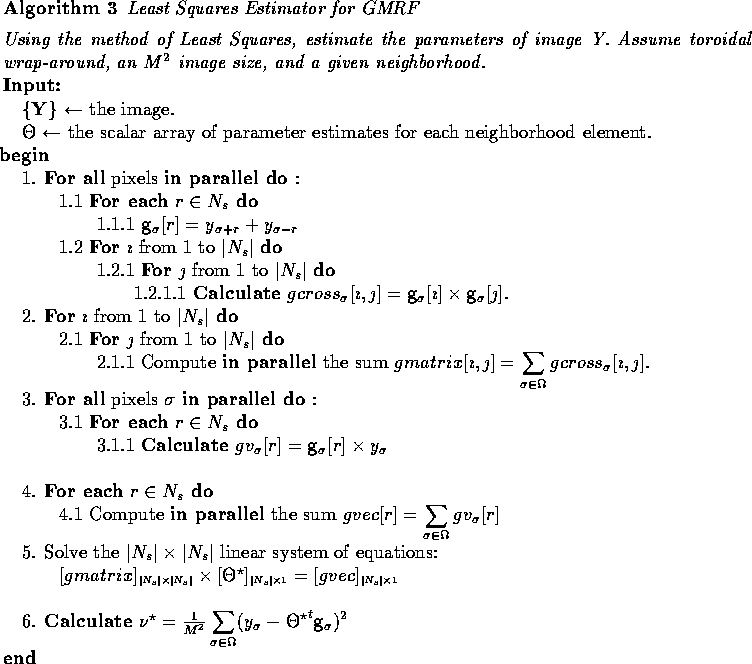For an image of size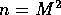, step 1.1 has a computational complexity of O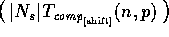parallel steps and a communication complexity of O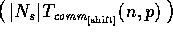. Step 1.2 runs in O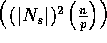parallel steps. Step 3 takes O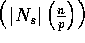parallel steps. Steps 2 and 4 contain a reduction over the entire array, specifically, finding the sum of the elements in a given parallel variable. As this is a scan operation, we refer the reader to Subsection 2.4\ for algorithm analysis. Thus, step 2 runs is O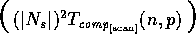computational parallel steps with O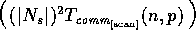communications, and steps 4 and 6 run in O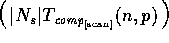parallel steps with O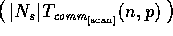communications. Solving the linear system of equations in step 5 takes O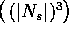computational steps.

The computational complexity of the Least Squares Estimator for an image of sizeis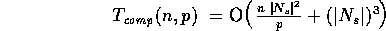and the communication complexity is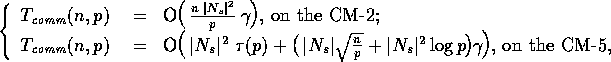using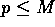processors.Next: Maximum Likelihood Estimate Up: Parameter Estimation for Previous: Parameter Estimation for